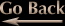Fractal Vector Fields
Ivan Freyman, 03/18/2010

Any point iterated with a fractal formula such as z=z2+c will have what is commonly referred to as an "orbit". It's the path a point takes as it hops from place to place on the complex plane. Sometimes these orbits will take the point to infinity; other times the orbits will steadily oscillate between several values, and another possibility is that the orbit will spiral into a fixed location. Regardless of what the orbit does, it will always have a specific magnitude and direction (vector) at each iteration. If we calculate the orbital vectors for a grid of points across any fractal in the complex plane, and we slowly change some variable in the formula for each frame of a movie, we will get a moving vector field. Below are the results of my programming experiments with this idea.

Vectors to Colors

As the title implies, instead of drawing an arrow we can draw a pixel whose color corresponds to the vector direction. The first two movies below map the vector direction to a standard HSV color wheel. The second movie focuses on the primary colors only (red, green, blue and cyan, magenta, yellow) while keeping all other pixels black so as to achieve a color banding effect. This creates sharp boundaries and makes it easier to see the wave-like motion (thanks to Carl Weiman for suggesting this coloring technique). The last movie is a morphing Julia set whose seed point traces a semicircle inside the top half of the Mandelbrot set's main body. It has nothing to do with vectors but it's still fun to watch.

Orbit Traps

If an orbit gets too close to one of the axes, iteration is halted and the currently iterated point is colored in proportion to the distance to the axis. This kind of trap produces straight stalks as seen in the image below on the left, but I wanted to create sinusoidal stalks. I've never seen it done before but figured it would look neat. Second from the left is a "zero" Julia set (the Julia constant is 0+0i) The others have constants (.32, .043i), (.32, .03822i), and (.32, .043i).

The picture on the far right does not have an orbit trap. Each pixel's color is based on the total distance traveled by a point's orbit after 71 iterations, provided the point does not escape to infinity. Points that escape are colored black.